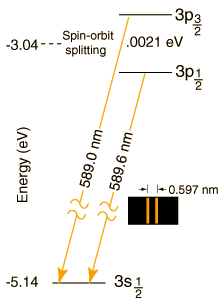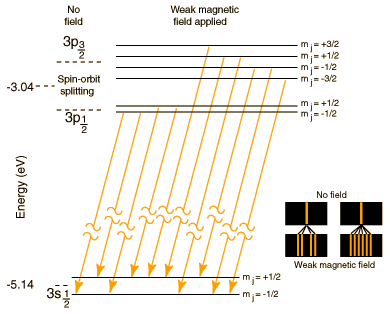# The Sodium DoubletThe well known bright doublet which is responsible for the bright yellow light from a sodium lamp may be used to demonstrate several of the influences which cause splitting of the emission lines of atomic spectra. The transition which gives rise to the doublet is from the 3p to the 3s level, levels which would be the same in the hydrogen atom. The fact that the 3s (orbital quantum number = 0) is lower than the 3p (l=1) is a good example of the dependence of atomic energy levels on angular momentum. The 3s electron penetrates the 1s shell more and is less effectively shielded than the 3p electron, so the 3s level is lower (more tightly bound). The fact that there is a doublet shows the smaller dependence of the atomic energy levels on the total angular momentum . The 3p level is split into states with total angular momentum j=3/2 and j=1/2 by the magnetic energy of the electron spin in the presence of the internal magnetic field caused by the orbital motion. This effect is called the spin-orbit effect. In the presence of an additional externally applied magnetic field, these levels are further split by the magnetic interaction, showing dependence of the energies on the z-component of the total angular momentum. This splitting gives the Zeeman effect for sodium.

The magnitude of the spin-orbit interaction has the form μzB = μBSzLz. In the case of the sodium doublet, the difference in energy for the 3p3/2 and 3p1/2 comes from a change of 1 unit in the spin orientation with the orbital part presumed to be the same. The change in energy is of the form

ΔE = μBgB = 0.0021 eV

where μB is the Bohr magneton and g is the electron spin g-factor with value very close to 2. This gives an estimate of the internal magnetic field needed to produce the observed splitting:

μBgB = (5.79 x 10-5 eV/T)2B = 0.0021 eV

B = 18 Tesla

This is a very large magnetic field by laboratory standards. Large magnets with dimensions over a meter, used for NMR and ESR experiments, have magnetic fields on the order of a Tesla.

 More on sodium spectrum Sodium Zeeman effect
Index

Other spectra

References
Thornton & Rex
Sec 9.2

Serway, Moses, Moyer
Sec 8.3

 HyperPhysics***** Quantum Physics R Nave
Go Back

# The Sodium Zeeman Effect

The sodium spectrum is dominated by the bright doublet known as the Sodium D-lines at 588.9950 and 589.5924 nanometers. From the energy level diagram it can be seen that these lines are emitted in a transition from the 3p to the 3s levels.The sodium doublet is further spit by the application of an external magnetic field ( Zeeman effect). Using the vector model for total angular momentum, the splitting is seen to produce one level for each possible value of the z-component of the total angular momentum J.

The size of the magnetic energy contribution depends upon a geometrical factor called the Lande' g-factor. The values for the relevant quantum numbers and the associated values for the Lande' g-factor are shown in the table below.

 Term J L S gL 3p3/2 3/2 1 1/2 4/3 3p1/2 1/2 1 1/2 2/3 3s1/2 1/2 0 1/2 2

Examination of the size of the Lande g-factor gL for the three levels will show why the splittings of the different levels are different in magnitude. The selection rules explain why the transitions shown are allowed and others not.

 Some history
Index

 HyperPhysics***** Quantum Physics R Nave
Go Back

# Sodium Zeeman Effect: Early Observations

The splitting of the sodium doublet in the presence of an external magnetic field was observed by Pieter Zeeman in 1896, and the effect was subsequently named the Zeeman effect. It is remarkable that so much detailed spectroscopy was done long before the Bohr theory, and perhaps even more remarkable that Zeeman's first study of the sodium Zeeman splitting was done the year before J. J.Thomson's discovery of the electron in 1897.

After Thomson's work, Zeeman and Lorentz did further study of the influence of magnetic fields on the spectral emissions from atoms. By analysis of the splitting of the sodium doublet, they were able to demonstrate that the charge to mass ratio of the charge responsible for the splitting was the same as Thomson's electron. This was the first direct demonstration that electrons were involved in the production of the spectral line emissions.

Index

Reference
Leighton
Ch 2

 HyperPhysics***** Quantum Physics R Nave
Go Back